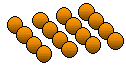Email us to get an instant 20% discount on highly effective K-12 Math & English kwizNET Programs!

#### Online Quiz (WorksheetABCD)

Questions Per Quiz = 2 4 6 8 10

### Grade 3 - Mathematics2.3 Practice Division with Pictures

 Method: Read the question. Count the pictures in each group. Write the number of items in one group or the number of groups Example: In the picture 9 items are divided into 3 groups with ___ in each.This is made of 3 groups of 4 in each. Answer : 4 Directions: Answer the following questions. Also write at least ten examples of your own.
 Q 1: In the picture below 8 rectangles are divided into 4 groups with ___ in each group.342 Q 2: In the picture below 6 stars are divided into 3 groups with ___ in each group.213 Q 3: In the picture below 9 boxes are divided into 3 groups with ___ in each group.364 Q 4: In the picture below 16 balls are divided into ___ groups with 4 balls in each group.5164 Q 5: In the picture below 12 fishes are divided into 4 groups with ___ in each group.364 Q 6: In the picture below 12 cylinders are divided into ___ groups with 4 cylinders in each group.436 Q 7: In the picture below _____ hearts are divided into 4 groups with 5 in each group.21202422 Q 8: In the picture below 8 medals are divided into 4 groups with ___ in each group.342 Question 9: This question is available to subscribers only! Question 10: This question is available to subscribers only!